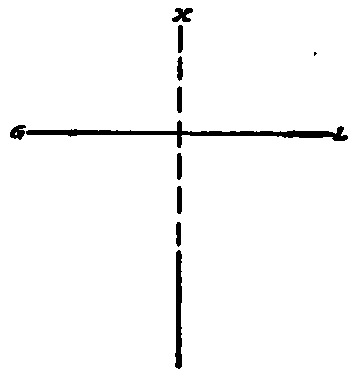## Principles

If a line is parallel to a plane, its projection on that plane will be equal in length to the line itself, as represented in Fig. 115. If a line is perpendicular to a plane, its projection on the plane will be a point, as represented by the cross in Fig. 116. If a line is inclined to a plane, its projection on that plane will be shorter than the line itself, as represented in Fig. 117. If a line is parallel to the horizontal or vertical plane, its projection on the other plane will be parallel to the ground line, as represented in Fig. 118. A line inclined to both the horizontal and vertical planes will not show its true length in either projection, as represented in Fig. 119. In a case like the one last mentioned, the true length of the line is found by revolving the line until it is parallel to one of the planes. Then, its projection on that plane will be its true length.Fig. 115. Projections of a Lino Parallel to Plane.Fig. 116. Projections of a Line Perpendicular to a Plane.Fig. 117. Projections of a Line Inclined to Plane.Fig. 118. Projections of Lines Parallel to Ground Line.

## True Length By Revolving Horizontal Projection

In Fig. 120 is shown the horizontal and vertical projections of the line AB, and to find the true length of the line itself proceed as follows: Swing the horizontal projection AhBh about one end Ah as a pivot, until it is parallel to the ground line. Project the new point B1h downward to a point on the vertical plane to a line drawn from B parallel to the ground line, locating the point B1,. The line connecting B1, and Av is the true length desired, since the true length of a line is always shown by its projection on a plane when the line is parallel to that plane.

## True Length By Revolving Vertical Projection

In Fig. 121 is shown the method of finding the true length of the same line as in Fig. 120, but by revolving the vertical projection. The method is the same. Revolve A'B' about the end Bv as a pivot until it is parallel to the ground line, and then project A1v up to A1h on the horizontal plane at the same distance from the ground line as Ah. The true length is then shown on the horizontal plane by the line connecting A1h and Bh. Projection lines representing the true length are always shown as dot and dash lines, as in Fig. 120 and 121.Fig. 119. True Length of Inclined Line not Shown in Its Projections.Fig. 120. True Length of a Line by Revolving Horizontal Projection.Fig. 121. True Length of a Line by Revolving Vertical Projection.